# de Branges' positivity condition

Let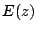be an entire function satisfying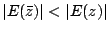for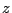in the upper half-plane. Define a Hilbert space of entire functions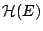to be the set of all entire functions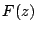such that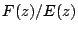is square integrable on the real axis and such that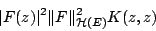for all complex, where the inner product of the space is given by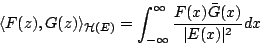for all elements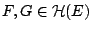and where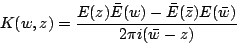is the reproducing kernel function of the space, that is, the identity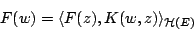holds for every complex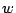and for every element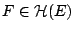.

de Branges [ MR 87m:11050] and [ MR 93f:46032] proved the following beautiful

Theorem . Letbe an entire function having no real zeros such thatfor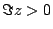, such that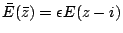for a constant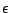of absolute value one, and such that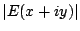is a strictly increasing function of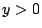for each fixed real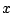. If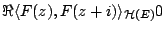for every element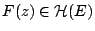with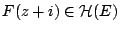, then the zeros oflie on the line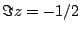, and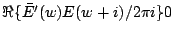whenis a zero of.

Let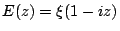. Then it can be shown that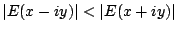for, and thatis a strictly increasing function of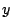on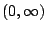for each fixed real. Therefore, it is natural to ask whether the Hilbert space of entire functionssatisfies the condition that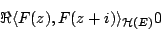for every elementofsuch that, because if so, then the Riemann Hypothesis would follow.

It is shown in [ MR 2001h:11114] that this condition is not satisfied.

Back to the main index for The Riemann Hypothesis.